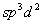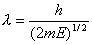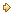Visitors Online: 77 | Monday 11th November 2019

CBSE Guess > Papers > Question Papers > Class XII > 2003 > Chemistry > Outside Delhi Set -II.

CHEMISTRY—2003 (Set II—Outside Delhi)

Note: Except for the following questions, all the remaining questions have been asked in Set I .

Q. 1. Write the composition of double-base propellant. 1

Q. 2. What is meant by inversion of sugar? 1

Q. 9. Name the radio active series which starts from Plutonium -241 and terminates at Bismuth-209. 1

Q. 10. Write a neutral molecule in which the central atom issp hybridized. 1

Q. 13. What is meant by specific conductivity of a solution?
The specific conductance of a 0.12 N solution of an electrolyte is 2.4 x 10 -2 S cm -1 . Calculate its equivalent conductance. 2

Q. 20. Write equations for the synthesis of the following: 2
(i) Glyptal
(ii) Neoprene

Q. 25. Illustrate the following with an example of reaction: 2
(i) Ambident nucleophile
(ii) Hinsberg test

Q. 27. Give appropriate reason for each of the following observations: 3
(i) Only higher members of Group 18 of the periodic table are expected to form compounds.
(ii) Fluorine is a stronger oxidising agent than chlorine, though fluorine has lower electron affinity than chlorine.
(ii) NO 2 readily forms a dimer, whereas CIO 2 does not.

Q. 28. Complete the following reactions: 3

(i) CH 3 - CH - CH 3 + PCI 5 -->

(ii)CH 3
|
(iii) CH 3 - C - X + NaOH -->
|
CH 3

Q. 29. What is meant by Vant's Hoff factor?
The osmotic pressure of a 0.0103 molar solution of an electrolyte is found to be 0.70 atm at 27 0 C. Calculate the Van't Hoff factor.
[R = 0.082 L atm-mol -1 K -1]
What conclusion do you draw about the molecular state of the solute in the solution?

Q. 32. Explain what is meant by dual nature of a particle in motion.
Show that the wavelength of a moving particle is related to its kinetic energy (E) as.

 Chemistry 2003 Question Papers Class XII Delhi Outside Delhi Compartment Delhi Compartment Outside DelhiSet ISet ISet ISet ISet IISet IISet IISet II

CBSE 2003 Question Papers Class XII